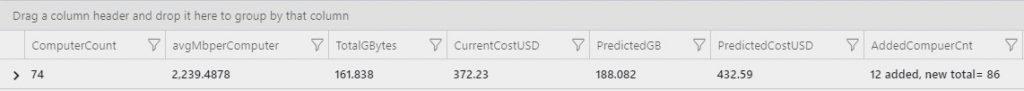# Azure Log Analytics: looking at data and costs – Part 4

You would probably take the data projection (see post 3) and add it into Excel to do the math, but you can also use KQL for that.

I assigned a price of \$2.30 (line 1); most of the rest of the syntax is the same. This is correct as of 22/7/2019, please check and adjust. There are references to USD in the summarize command, if you do use a local currency there are two changes to make.

``` // // How much would it cost if I added an extra nn Computers? // Only looks at the billable data // // You can add 2 options, // // 1. A count of the extra computers to add // 2. Count of days to look back at, to get historical data - suggested 31days // 3. USD \$ price // All prices shown are in US Dollar (\$). This is a summary estimate, not a quote. For up to date pricing information please visit https://azure.microsoft.com/pricing/calculator/ ```

let aap = 2.30; //Add Azure Pricing (\$ USD) source: https://azure.microsoft.com/en-us/pricing/details/monitor/
let daystoSearch = 31d;
union withsource = tt *
| where TimeGenerated > startofday(ago(daystoSearch))
| where _IsBillable == true
| where isnotempty(Computer)
| summarize
ComputerCount = dcount(Computer),
avgMbperComputer= round(sum(_BilledSize / (1024*1024)) / dcount(Computer),4),
TotalGBytes = round(sum(_BilledSize / (1024*1024*1024)),4),
CurrentCostUSD = round(sum(_BilledSize / (1024*1024*1024)) * aap,2),
PredictedGB = round(sum(_BilledSize / (1024*1024*1024)) / dcount(Computer) * (dcount(Computer) + addExtraComputers),4),
PredictedCostUSD= round(sum(_BilledSize / (1024*1024*1024)) / dcount(Computer) * (dcount(Computer) + addExtraComputers) * aap,2),